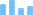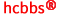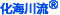##HCBBS 长度单位换算

 长度换算   ←【加入收藏夹(Add to Favorites)】 米(m) 千米(km) 分米(dm) 厘米(cm) 毫米(cm) 微米(cm) 里 丈 尺 寸 分 厘 英尺(ft) 英寸(in) 英里(mi) 海里(nmi)

 长 度 换 算 1千米(km)= 0.621英里(mile) 1米(m)= 3.281英尺(ft)= 1.094码(yd) 1丝米(dmm)=1忽米(cmm)=1丝=0.01毫米=0.001厘米 1厘米(cm)= 0.394英寸(in) 1厘米（cm)=10毫米（mm) 10^9纳米 = 1米（m） 10^6纳米 = 1毫米（mm） 10^3纳米 = 1微米（μm） 1纳米 = 10 埃米（记为Å） 1埃米=10^-10m 1埃(Å)= 0.1纳米(nm) 1英里(mile)= 1.609千米(km) 1英寻(fm)= 1.829米(m) 1英尺(ft)= 0.3048米(m) 1英寸(in)= 2.54厘米(cm) 1海里(n mile)= 1.852千米(km) 1链= 66英尺(ft)= 20.1168米 1码(yd)= 0.9144米(m) 1密耳(mil)= 0.0254毫米(mm) 1英尺(ft)= 12英寸(in) 1码(yd)= 3英尺(ft) 1杆(rad)= 16.5英尺(ft) 1英里(mile)= 5280英尺(ft) 1海里(n mile)= 1.1516英里(mile) 市制计量单位： 1里=150丈 1丈=10尺 1尺=10寸 1寸=10分 1分=10厘 1厘=10毫

 或 海川化工论坛微信公众号底部菜单入口 或 手机浏览或微信扫描下码进入手机版荣誉贡献单： 化海川流 流星723 提供校对支持、比率统计 20210908 化海川流社区  清洁工 提供界面设计，项目管理 20210901 化海川流社区  cookye 、henglinkou    提供数据支持 20210909 本项目扩展支持，可联系 电子邮箱 service@hcbbs.com  或使用海川化工论坛站内信 联系 清洁工 管理员。

 客服电话18840911640(微信同号)   0411-88254066      会员服务:电子邮箱 service@hcbbs.com(客服信箱)                    商务合作:GMT+8, 2022-9-29 18:29   平台统计：化海川流(hcbbs.com) Copyright By,0.026060 second(s), 9 queries , Redis On.